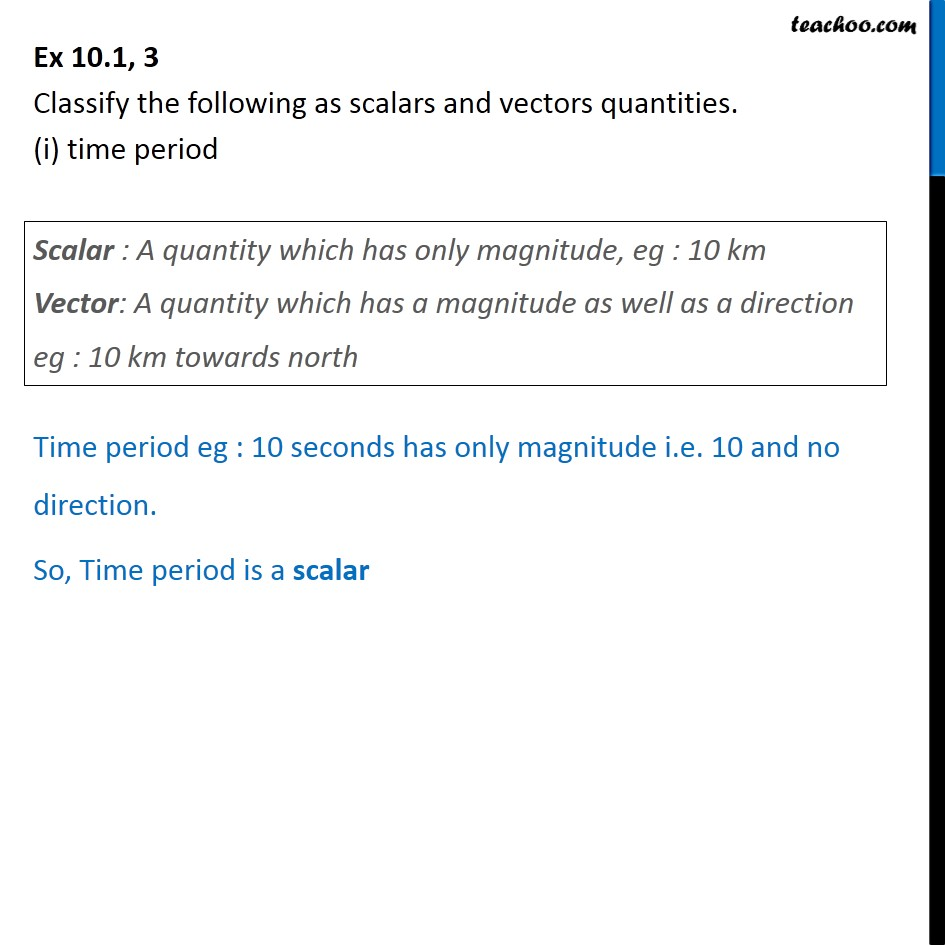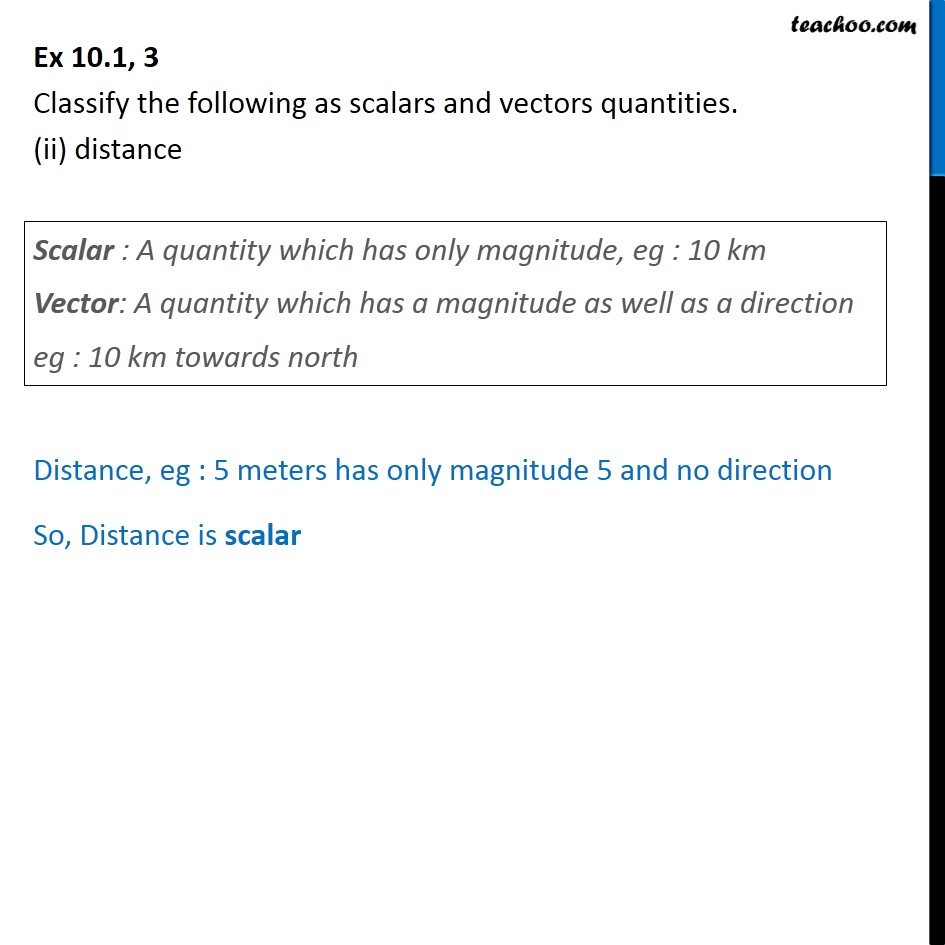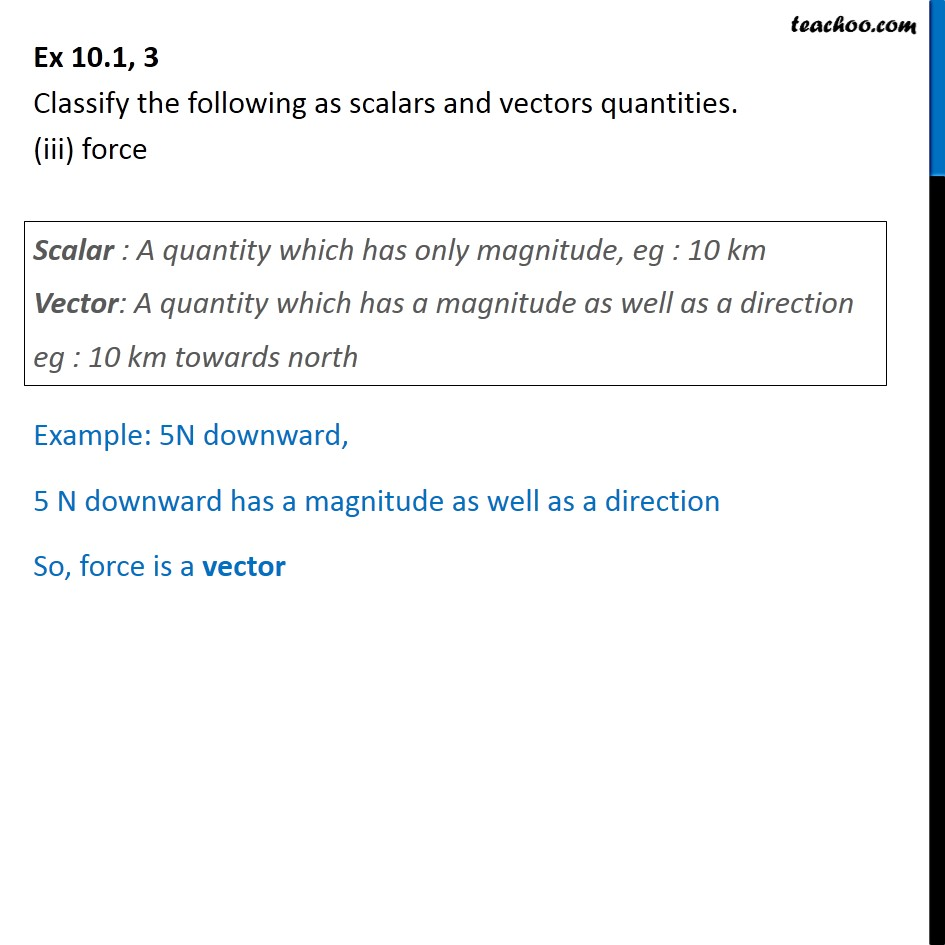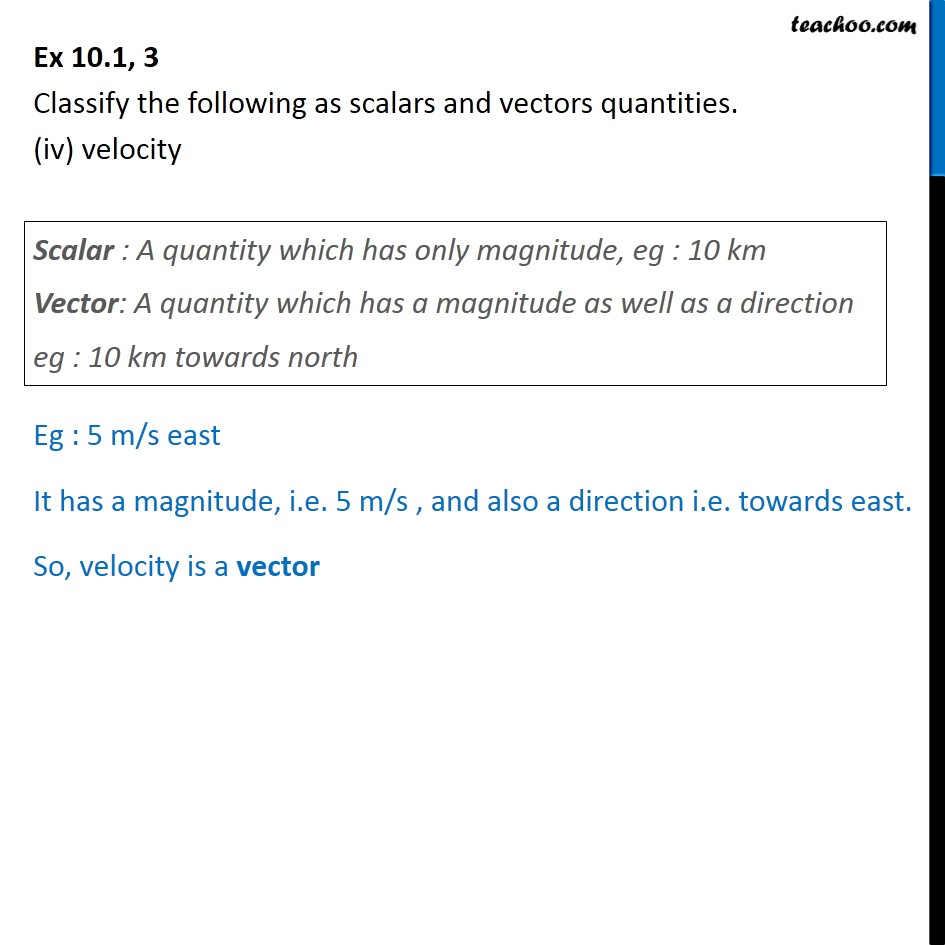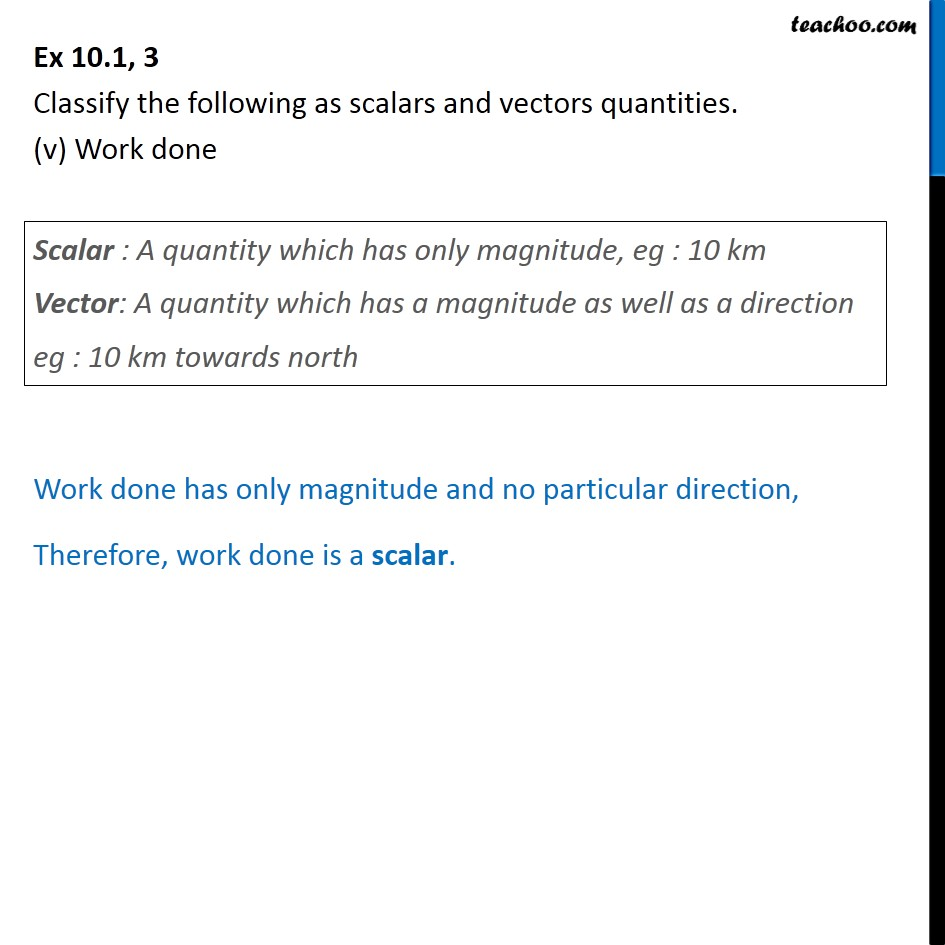Subscribe to our Youtube Channel - https://you.tube/teachoo

1. Chapter 10 Class 12 Vector Algebra
2. Concept wise
3. Scalar or vector

Transcript

Ex 10.1, 3 Classify the following as scalars and vectors quantities. (i) time period Time period eg : 10 seconds has only magnitude i.e. 10 and no direction. So, Time period is a scalar Ex 10.1, 3 Classify the following as scalars and vectors quantities. (ii) distance Distance, eg : 5 meters has only magnitude 5 and no direction So, Distance is scalar Ex 10.1, 3 Classify the following as scalars and vectors quantities. (iii) force Example: 5N downward, 5 N downward has a magnitude as well as a direction So, force is a vector Ex 10.1, 3 Classify the following as scalars and vectors quantities. (iv) velocity Eg : 5 m/s east It has a magnitude, i.e. 5 m/s , and also a direction i.e. towards east. So, velocity is a vector Ex 10.1, 3 Classify the following as scalars and vectors quantities. (v) Work done Work done has only magnitude and no particular direction, Therefore, work done is a scalar.

Scalar or vector

About the AuthorDavneet Singh
Davneet Singh is a graduate from Indian Institute of Technology, Kanpur. He has been teaching from the past 9 years. He provides courses for Maths and Science at Teachoo.